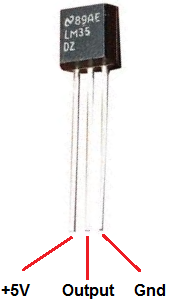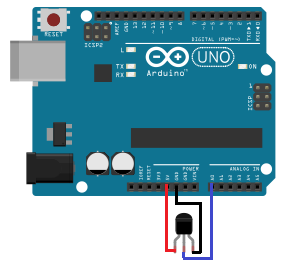﻿ How to Build a LM35 Temperature Sensor Circuit ﻿# How to Build a LM35 Temperature Sensor CircuitIn this project, we will demonstrate how to build temperature sensor circuit using a LM35 sensor.

As a temperature sensor, the circuit will read the temperature of the surrounding environment and relay thi temperature to us back in degrees celsius.

The IC we will use to measure the temperature is the LM35 IC. We will integrate this with the arduino to measure the temperature. The arduino will then read this measured value from the LM35 and translate into degrees fahrenheit and celsius, which we will be able to read from the computer from the arduino serial monitor.

Components Needed to Build the LM35 Temperature Sensor Circuit

• Arduino Board
• LM35 Temperature Sensor IC
• Computer
• USB with type A and B connectors

We can use any type of arduino board.

The LM35 is a low voltage IC which uses approximately +5VDC of power. This is ideal because the arduino's power pin gives out 5V of power. The IC has just 3 pins, 2 for the power supply and one for the analog output.

The output pin provides an analog voltage output that is linearly proportional to the celsius (centigrade) temperature. Pin 2 gives an output of 1 millivolt per 0.1°C (10mV per degree). oSo to get the degree value in celsius, all that must be done is to take the voltage output and divide it by 10- this give out the value degrees in celsius.

So, for example, if the output pin, pin 2, gives out a value of 315mV (0.315V), this is equivalent to a temperature of 31.5°C.

We can then easily convert this celsius value into fahrenheit by plugging in the appropriate conversion equation. All we must do is write this code and upload it to the arduino to convert this celsius temperature into fahrenheit. The code is shown below.

Below is the pinout of the LM35 IC:Pin 1 receives positive DC voltage in order for the IC to work. This, again, is voltage approximately 5 volts. Pin 3 is the ground, so it receives the ground or negative terminal of the DC power supply. And Pin 2 is the output of the IC, outputting an analog voltage in porportion to the temperature it measures.

This is the datasheet of the LM35 IC: LM35 Temperature Sensor IC Datasheet.

The arduino, with suitable code, can then interpret this measured analog voltage and output to us the temperature in degrees celsius and fahrenheit.

Also to do this project we need a USB cable with a Type A connector on one end and a Type B connector on the other end. This is so that we can hook our arduino to a computer and send it code that it can run to display to us the temperature.

### Temperature Sensor Circuit Schematic

The temperature sensor circuit we will build is shown below:This translates into the circuit schematic:So you circuit connections are:

Pin 1 of the LM35 goes into +5V of the arduino
Pin 2 of the LM35 goes into analog pin A0 of the arduino
Pin 3 of the LM35 goes into ground (GND) of the arduino

Now that we have this circuit setup, we now connect the USB cable from the arduino to the computer. The type B side of the connector goes into the arduino and the type A side into the USB port of the computer. Now the computer is connected to the arduino. We can now write code in the processing software to give instructions to the arduino.

### Code for Temperature Sensor Circuit

//initializes/defines the output pin of the LM35 temperature sensor
int outputpin= 0;
//this sets the ground pin to LOW and the input voltage pin to high
void setup()
{
Serial.begin(9600);
}

//main loop
void loop()
{
float millivolts= (rawvoltage/1024.0) * 5000;
float celsius= millivolts/10;
Serial.print(celsius);
Serial.print(" degrees Celsius, ");

Serial.print((celsius * 9)/5 + 32);
Serial.println(" degrees Fahrenheit");
delay(1000);

}

The code will now be explained. Before we can get a celsius reading of the temperature, the analog output voltage must first be read. This will be the raw value divided by 1024 times 5000. It is divided by 1024 because a span of 1024 occupies 5V. We get the ratio of the raw value to the full span of 1024 and then multiply it by 5000 to get the millivolt value. Since the output pin can give out a maximum of 5 volts (1024), 1024 represents the possible range it can give out. The raw voltage over this 1024 (value) therefore represents the ratio of how much power the output pin is outputting against this full range. Once we have this ratio, we then multiply it by 5000 to give the millivolt value. This is because there is 5000 millvolts in 5 volts.

Once this analog voltage in millivolts is calculated, we then can find the temperature in fahrenheit by the equation: ((celsius * 9)/5 + 32)

At the end of this program, we put a delay of 5000ms to take the temperature reading every 5 seconds. You can adjust this value to meet your personal preference or program needs.

To see this circuit in action, watch the following video below.

To see how to integrate this circuit to an LCD for an LCD readout instead of from the Serial Monitor, see How to Integrate a Temperature Sensor Circuit to an LCD.

Related Resources